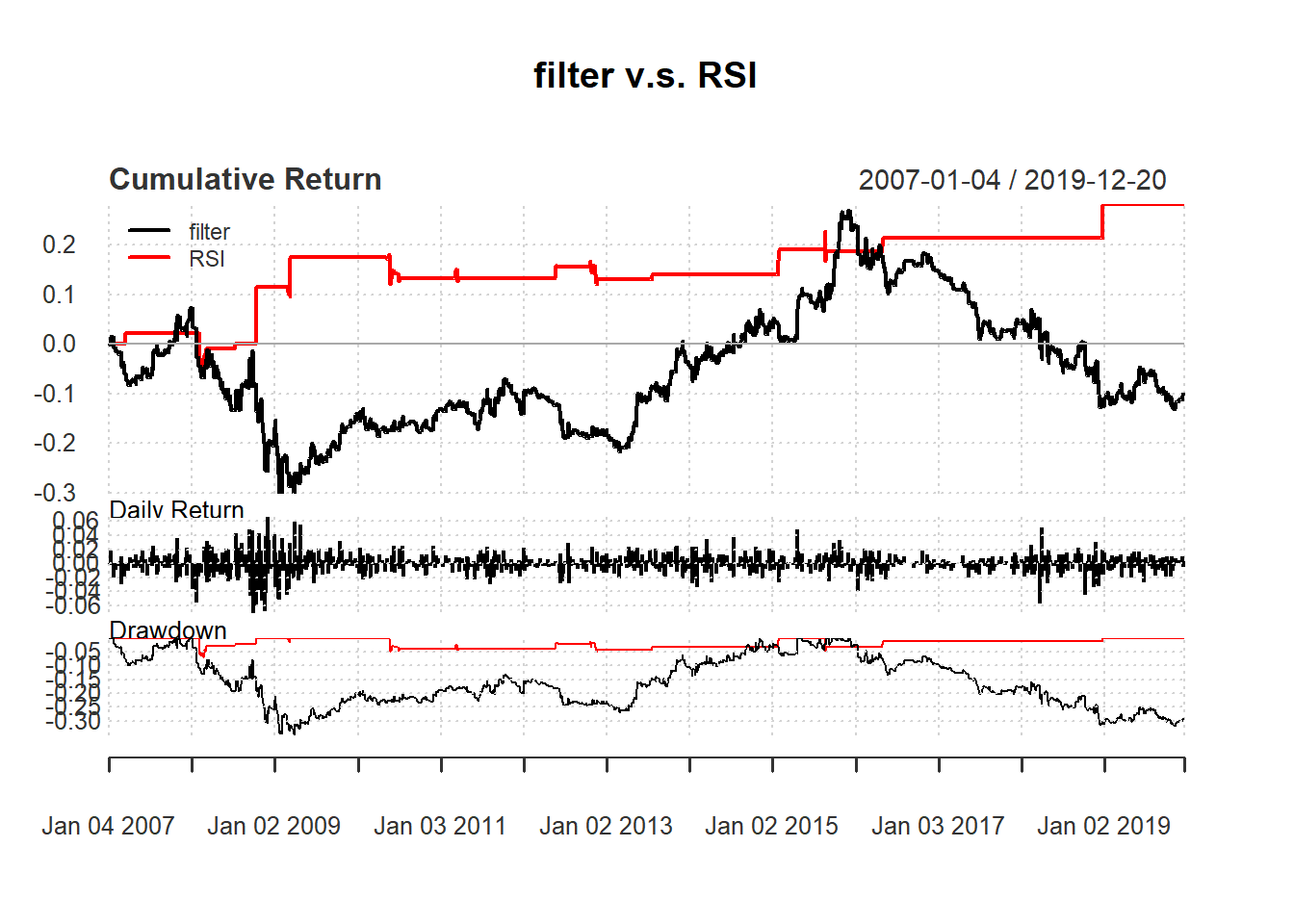## 7.6 Comparing rules

We can compare filter and RSI rules directly.

day <-14
price <- Cl(MSFT)
returnMSFT <- (Cl(MSFT) - Op(MSFT))/Op(MSFT)

r <- price/Lag(price) - 1 # % price change
delta <-0.005 #threshold
filter.signal <-c(0) # first date has no signal

rsi <- RSI(price, day)     #rsi is the lag of RSI
RSI.signal <- c()          #initialize vector
RSI.signal [1:day+1] <- 0  #0 because no signal until day+1

We calculate the trading signal for filter rule:

for (i in 2: length(price)){
if (r[i] > delta){
filter.signal[i]<- 1
} else
filter.signal[i]<- 0
}
filter.signal<-reclass(filter.signal,Cl(MSFT))

We calculate the trading signal for RSI rule:

for (i in (day+1): length(price)){
if (rsi[i] < 30){             #buy if rsi < 30
RSI.signal[i] <- 1
}else {                       #no trade all if rsi > 30
RSI.signal[i] <- 0
}
}

RSI.signal<-reclass(RSI.signal,Cl(MSFT))

We now all apply our trading rules are based on yesterday signal:

filter.trade <- Lag(filter.signal,1)
names(RSI.ret) <- 'RSI'
retall <- cbind(filter.ret, RSI.ret)
main="filter v.s. RSI")